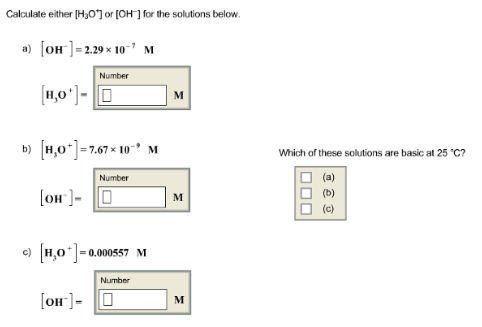# Problem: Calculate either [H3O+] or [OH -] for the solution below. Which of these solutions are basic at 25°C?

###### FREE Expert Solution###### Problem Details

Calculate either [H3O+] or [OH -] for the solution below.

Which of these solutions are basic at 25°C?